Next: MHD Waves Up: Magnetohydrodynamic Fluids Previous: Magnetic Pressure

# Flux Freezing

The MHD Ohm's law,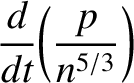(693)

is sometimes referred to as the perfect conductivity equation, for obvious reasons, and sometimes as the flux freezing equation. The latter nomenclature comes about because Eq. (693) implies that the magnetic flux through any closed contour in the plasma, each element of which moves with the local plasma velocity, is a conserved quantity.

In order to verify the above assertion, let us consider the magnetic flux,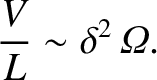, through a contour,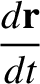, which is co-moving with the plasma:(694)

Here,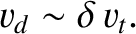is some surface which spans. The time rate of change ofis made up of two parts. Firstly, there is the part due to the time variation of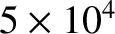over the surface. This can be written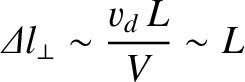(695)

Using the Faraday-Maxwell equation, this reduces to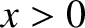(696)

Secondly, there is the part due to the motion of. If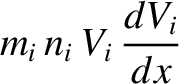is an element ofthen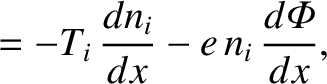is the area swept out byper unit time. Hence, the flux crossing this area is. It follows that(697)

Using Stokes's theorem, we obtain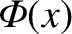(698)

Hence, the total time rate of change ofis given by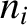(699)

The condition(700)

clearly implies thatremains constant in time for any arbitrary contour. This, in turn, implies that magnetic field-lines must move with the plasma. In other words, the field-lines are frozen into the plasma.

A flux-tube is defined as a topologically cylindrical volume whose sides are defined by magnetic field-lines. Suppose that, at some initial time, a flux-tube is embedded in the plasma. According to the flux-freezing constraint,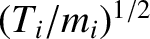(701)

the subsequent motion of the plasma and the magnetic field is always such as to maintain the integrity of the flux-tube. Since magnetic field-lines can be regarded as infinitely thin flux-tubes, we conclude that MHD plasma motion also maintains the integrity of field-lines. In other words, magnetic field-lines embedded in an MHD plasma can never break and reconnect: i.e., MHD forbids any change in topology of the field-lines. It turns out that this is an extremely restrictive constraint. Later on, we shall discuss situations in which this constraint is relaxed.Next: MHD Waves Up: Magnetohydrodynamic Fluids Previous: Magnetic Pressure
Richard Fitzpatrick 2011-03-31